# PSEB 11th Class Maths Solutions Chapter 6 Linear Inequalities Ex 6.1

Punjab State Board PSEB 11th Class Maths Book Solutions Chapter 6 Linear Inequalities Ex 6.1 Textbook  Exercise Questions and Answers.

## PSEB Solutions for Class 11 Maths Chapter 6 Linear Inequalities Ex 6.1

Question 1.
Solve 24 x < 100, when
(i) x is a natural number
(ii) x is an integer.
We have, 24 x < 100
⇒ $$\frac{24 x}{24}<\frac{100}{24}$$ [dividing bothsides by 24]

⇒ x < $$\frac{25}{6}$$

(i) When x is a natural number, then solutions of the inequality are given by x < $$\frac{25}{6}$$ i.e., all natural numbers x which are less than $$\frac{25}{6}$$.

In this case, the following values of x make the statement true. x = 1, 2, 3, 4
Hence, the solution set of inequality is {1, 2, 3, 4}.

(ii) When x is an integer. In this case, solutions of given inequality are …, – 4, – 3, – 2, – 1, 0, 1, 2, 3, 4. Hence, the solution set of inequality is {…, – 4, – 3, – 2, – 1, 0, 1, 2, 3, 4}.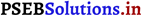Question 2.
Solve 12x > 30, when
(i) x is a natural number
(ii) x is an integer.
The given inequality is – 12x > 30.
$$\frac{-12 x}{-12}<\frac{30}{-12}$$ [dividing bothsides by 24]
⇒ x < – $$\frac{5}{2}$$

(i) There is no natural number less than (- $$\frac{5}{2}$$) are …………. – 5, – 4, – 3.
Thus, when x is a natural number, there is no solution of the given inequality.

(ii) The integers less than (- $$\frac{5}{2}$$) are ………. – 5, – 4, – 3.
Thus, when x is an integer, the solutions of the given inequality are ………….., – 5, – 4, – 3.
Hence, in this case, the solution set is {………, – 5, – 4, – 3}.Question 3.
Solve 5x – 3 < 7, when
(i) x is an integer
(ii) x is a real number.
We have,
5x – 3 < 7
⇒ 5x – 3 + 3 < 7 + 3 [adding 3 on bothsides]
⇒ 5x < 10
∴ x < 2 [dividing both sides by 5]
Thus, ‘any number less than 2 satisfies given inequality.

(i) When x is an integer, then the solution of the given inequality is (…., – 4, – 3, – 2, – 1, 0, 1}.

(ii) When x is a real number then the solution of the inequality is given by
x < 2 i.e.,aIl real numbers x,which are less than 2. Hence, solution set is (- ∞, 2). Question 4. Solve 3x + 8 > 2, when
(i) x is an integer
(ii) x is a real number.
The given inequality is 3x + 8 > 2.
3x + 8 > 2
⇒ 3x + 8 – 8 > 2 – 8 = 3x > – 6
⇒ 3x > – 6
⇒ $$\frac{3 x}{3}>\frac{-6}{3}$$
⇒ x > – 2

(i) The integers greater than – 2 are – 1, 0, 1, 2, …………
Thus, when x is an integer, the solutions of the given inequality are – 1, 0, 1, 2 …
Hence, in this case, the solution set is {- 1, 0, 1, 2, ……..}.

(ii) When x is a real number, the solutions of the given inequality are all the real numbers, which are greater than – 2.
Thus, in this case, the solutioñ set is (- 2, ∞).Question 5.
Solve the given inequality for real x : 4x + 3 < 5x + 7.
4x + 3 < 5x + 7
⇒ 4x + 3 – 7 < 5x + 7 – 7 z
⇒ 4x – 4 < 5x
⇒ 4x – 4 – 4x < 5x – 4x
⇒ – 4 < x Thus, all real numbers x, which are greater than – 4, are the solutions of the given inequality. Hence, the solution set of the given inequality is (- 4, ∞).

Question 6.
Solve the given inequality for real x : 3x – 7 > 5x – 1.
3x – 7 > 5x – 1
⇒ 3x – 7 + 7 > 5x – 1 + 7
⇒ 3x > 5x + 6
⇒ x – 5x > 5x + 6 – 5x
⇒ – 2x > 6
⇒ $$\frac{-2 x}{-2}<\frac{6}{-2}$$
⇒ 3x < 2x – 3
⇒ 3x – 2x < 2x – 3 – 2x
⇒ x < – 3. Thus, all real numbers x, which are less than or equal to – 3, are the solutions of the given inequality. Hence, the solution set of the given inequality is (- ∞, – 3].

Question 7.
Solve the given inequality for real x : 3(x – 1) ≤ 2 (x – 3).
3(x – 1) ≤ 2 (x – 3)
⇒ 3x – 3 ≤ 2x – 6
⇒ 3x – 3 + 3 ≤ 2x – 6 + 3
⇒ 3x ≤ 2x – 3
⇒ 3x – 2x ≤ 2x – 3 – 2x
⇒ x ≤ – 3.
Thus, all real numbers x, which are less than or equal to – 3, are the solutions of the given inequality.
Hence, the solution set of the given inequality is (- ∞, – 3].

Question 8.
Solve the given inequality for real x : 3(2 – x) > 2 (1 – x).
3 (2 – x) > 2 (1 – x)
⇒ 6 – 3x > 2 – 2x
⇒ 6 – 3x + 2x > 2 – 2x + 2x
⇒ 6 – x >2
⇒ 6 – x – 6 > 2 – 6
⇒ – x > – 4
⇒ x < 4.
Thus, all real numbers x,which are less than or equal to 4, are the solutions of the given inequality.
Hence, the solution set of the given inequality is (- ∞, 4].Question 9.
Solve the given inequality for real x : x + $$\frac{x}{2}+\frac{x}{3}$$ < 11.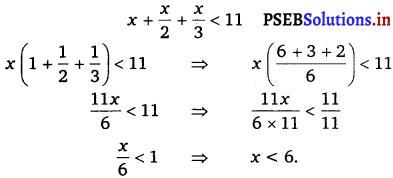Thus, all real numbers x, which are less than 6, are the solutions of the given inequality.
Hence, the solution set of the given inequality is (- ∞, 6).

Question 10. Solve the given inequality for real x : $$\frac{x}{3}>\frac{x}{2}$$+ 1.
$$\frac{x}{3}>\frac{x}{2}$$ + 1

⇒ $$\frac{x}{3}$$ – $$\frac{x}{2}$$ > 1

⇒ $$\frac{2 x-3 x}{6}$$ > 1

⇒ $$-\frac{x}{6}$$ > 1

⇒ – x > 6
⇒ x < – 6.
Thus, all real numbers x, which are less than – 6, are the solutions of the given inequality.
Hence, the solution set of the given inequality is (- ∞, – 6).

Question 11.
Solve the given inequality for real x :
$$\frac{3(x-2)}{5} \leq \frac{5(2-x)}{3}$$
$$\frac{3(x-2)}{5} \leq \frac{5(2-x)}{3}$$
⇒ 9(x – 2) ≤ 25 (2 – x)
⇒ 9x – 18 ≤ 50 – 25x
⇒ 9x – 18 + 25x ≤ 50
⇒ 34x – 18 ≤ 50
⇒ 34x ≤ 50 + 18
⇒ 34x ≤ 68
⇒ $$\frac{34 x}{34} \leq \frac{68}{34}$$
⇒ x ≤ 2
Thus, all real numbers x, which are less than or equal to 2, are the solutions of the given inequality.
Hence, the solution set of the given inequality is (- ∞, 2].Question 12.
Solve the given inequality for real x: x : $$\frac{1}{2}\left(\frac{3 x}{5}+4\right) \geq \frac{1}{3}(x-6)$$.
We have, $$\frac{1}{2}\left(\frac{3 x}{5}+4\right) \geq \frac{1}{3}(x-6)$$

⇒ $$\frac{1}{2}\left(\frac{3 x+20}{5}\right) \geq \frac{1}{3}(x-6)$$

⇒ $$\frac{1}{10}$$ (3x + 20) ≥ $$\frac{1}{3}$$ (x – 6)

⇒ $$\frac{30}{10}$$ (3x + 20) ≥ $$\frac{30}{3}$$ (x – 6) [multiplying both sides by 30]
⇒ 3 (3x + 20) ≥ 10 (x – 6)
⇒ 9x + 60 ≥ 10x – 60
⇒ 9x + 60 – 60 ≥ 10x – 60 – 60 [subtracting 60 from both sides]
⇒ 9x ≥ 10x – 120
⇒ 9x – 10x ≥ 10x -120 – 10x [subtracting 10x from both sides]
⇒ – x ≥ -120
⇒ x ≤ 120 [multiplying both sides by -1]
∴ x ∈ (- ∞, 120]
On number line, it can be represented as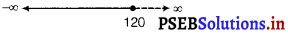Hence, the dark portion on the number line represents the solution.

Question 13.
Solve the given inequality for real x :
2(2x + 3) – 10 < 6 (x – 2)
2 (2x + 3) – 10 < 6 (x – 2)
⇒ 4x + 6 – 10 < 6x – 12
⇒ 4x – 4 < 6x – 12
⇒ – 4 + 12 < 6x – 4x
⇒ 8 < 2x
⇒ 4 < x. Thus, all real numbers x, which are greater than 4, are the solutions of the given inequality. Hence, the solution set of the given inequality is (4, ∞).Question 14.
Solve the given inequality for real x : 37 – (3x + 5) ≥ 9x – 8 (x – 3).
37 – (3x + 5) ≥ 9x – 8 (x – 3)
⇒ 37 – 3x – 5 ≥ 9x – 8x + 24
⇒ 32 – 3x ≥ x + 24
⇒ 32 – 24 ≥ x + 3x
⇒ 8 > 4x
⇒ 2 ≥ x.
Thus, all real numbers x, which are less than or equal to 2, are the solutions of the given inequality.
Hence, the solution set of the given inequality is (- ∞, 2].

Question 15.
Solve the given inequality for real x : $$\frac{x}{4}<\frac{(5 x-2)}{3}-\frac{(7 x-3)}{5}$$.

$$\frac{x}{4}<\frac{(5 x-2)}{3}-\frac{(7 x-3)}{5}$$

⇒ $$\frac{x}{4}<\frac{5(5 x-2)-3(7 x-3)}{15}$$

⇒ $$\frac{x}{4}<\frac{25 x-10-21 x+9}{15}$$

⇒ $$\frac{x}{4}<\frac{4 x-1}{15}$$

⇒ 15x < 4 (4x – 1)
⇒ 15x < 16x – 4
⇒ 4 < 16x – 15x
⇒ 4 < x
Thus, all real numbers x, which are greater than 4, are the solutions of the given inequality.
Hence, the solution set of the given inequality is (4, x).Question 16.
Solve the given inequality for real x: $$\frac{(2 x-1)}{3} \geq \frac{(3 x-2)}{4}-\frac{(2-x)}{5}$$
$$\frac{(2 x-1)}{3} \geq \frac{(3 x-2)}{4}-\frac{(2-x)}{5}$$

⇒ $$\frac{(2 x-1)}{3} \geq \frac{5(3 x-2)-4(2-x)}{20}$$

⇒ $$\frac{(2 x-1)}{3} \geq \frac{15 x-10-8+4 x}{20}$$

⇒ $$\frac{(2 x-1)}{3} \geq \frac{19 x-18}{20}$$

⇒ 20 (2x – 1) ≥ 3 (19x – 18)
⇒ 40x – 20 ≥ 57x – 54
⇒ – 20 + 54 ≥ 57x – 40x
⇒ 34 ≥ 17x
⇒ 2 ≥ x.
Thus, all real numbers x, which are less than or equal to 2, are the solutions of the given inequality.
Hence, the solution set of the given inequality is (- ∞, 2].

Question 17.
Solve the given inequality and show the graph of the solution on number line: 3x – 2 < 2x + 1.
3x – 2 < 2x + 1
⇒ 3x – 2x < 1 + 2
⇒ x < 3
The graphical representation of the solutions of the given inequality is as follows.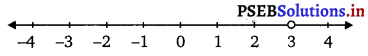All the numbers on the left side of 3 will be greather than it.
Hence, solution set of the given inequality is (- ∞, 3).Question 18.
Solve the given inequality and show the graph of the solution on number line: 5x – 3 ≥ 3x – 5.
5x – 3 ≥ 3x – 5
⇒ 5x – 3x ≥ – 5 + 3
⇒ 2x ≥ – 2
⇒ $$\frac{2 x}{2} \geq \frac{-2}{2}$$
⇒ – x ≥ – 1.
The graphical representation of the solutions of the given inequality is as follows.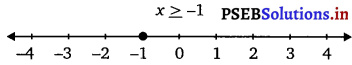All the numbers on the right side of – 1 will be greather than it.
Hence, solution set of the given inequality is [- 1, ∞].

Question 19.
Solve the given inequality and show the graph of the solution on number line : 3 (1 – x) < 2 (x + 4).
3 (1 – x) < 2 (x + 4)
⇒ 3 – 3x < 2x + 8
⇒ 3 – 8 < 2x + 3x
⇒ – 5 < 5x
⇒ $$\frac{-5}{5}<\frac{5 x}{5}$$
⇒ – 1 < x.
The graphical representation of the solutions of the given inequality is as follows.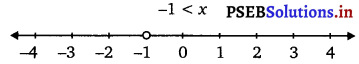Hence, solution set of the given inequality is (- 1, ∞).Question 20.
Solve the given inequality and show the graph of the solution on number line : $$\frac{x}{2} \geq \frac{(5 x-2)}{3}-\frac{(7 x-3)}{5}$$

$$\frac{x}{2} \geq \frac{(5 x-2)}{3}-\frac{(7 x-3)}{5}$$ $$\frac{x}{2} \geq \frac{5(5 x-2)-3(7 x-3)}{15} \Rightarrow$$ $$\frac{x}{2} \geq \frac{25 x-10-21 x+9}{15}$$

$$\frac{x}{2} \geq \frac{4 x-1}{15}$$
⇒ 15x ≥ 2 (4x – 1)
⇒ 15x ≥ 8x – 2
⇒ 7x ≥ – 2
⇒ x ≥ $$-\frac{2}{7}$$

The graphical representation of the solutions of the given inequality is as follows.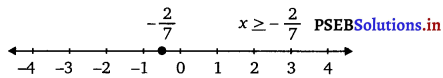Hence, solution set of the given inequality is [$$-\frac{2}{7}$$, ∞).

Question 21.
Ravi obtained 70 and 75 marks in first two unit test. Find the minimum marks he should get in the third test to have an average of at least 60 marks.
Let x be the marks obtained by Ravi in the third unit test.
Since the student should have an average of at least 60 marks,
$$\frac{70+75+x}{3}$$ ≥ 60
⇒ 145 + x ≥ 180
⇒ x ≥ 180 – 145
⇒ x ≥ 35.
Thus, the student must obtain a minimum of 35 marks to have an average of at least 60 marks.Question 22.
To receive Grade ‘A’ in a course, one must obtain an average of 90 marks or more in five examinations (each of 100 marks). If Sunita’s marks in first four examinations are 87, 92, 94 and 95, find minimum marks that Sunita must obtain in fifth examination to get grade ‘A’ in the course.
Let Sunita obtains x marks in her fifth examination.
Thus,  ≥ 90
⇒ 368 + x ≥ 450
⇒ 368 + x – 368 ≥ 450 – 368 [subtracting 368 from both sides]
∴ x ≥ 82
Thus, Sunita must obtain a minium of 82 marks to get grade A in the course.

Question 23.
Find all pairs of consecutive odd positive integers both of which are smaller than 10 such that their sum is more than 11.
Let x be the smaller of the two consecutive odd positive integers.
Then, the other integer is x + 2.
Since both the integers are smaller than 10,
x + 2 < 10
⇒ x < 10 – 2
⇒ x < 8 Also, the sum of the two integers is more than 11. x + (x + 2) > 11
⇒ 2x + 2 > 11
⇒ 2x > 11 – 2
⇒ 2x > 9
⇒ x > $$\frac{9}{2}$$
⇒ x > 45 ……………(ii)
From eqs. (i) and (ii), we obtain
Since x is an odd number, x can take the values, 5 and 7.
Thus, the required possible pairs are (5, 7) and (7, 9).Question 24.
Find all pairs of consecutive even positive integers, both of which are larger than 5, such that their sum is less than 23.
Let x be the smaller of the two positive even integers then the other one is x + 2, then we should have
x > 5 ……………(i)
and x + x + 2 < 23
or 2x + 2 < 23.
Since if the sum is less than 23, then it is also less than 24
2x + 2 < 24 .
or 2x < 22
or x < 11. Thus, the value of x may be 6, 8, 10 (even integer).
Hence, the pairs maybe (6, 8), (8, 10), (10, 12).

Question 25.
The longest side of a triangle is 3 times the shortest side and the third side is 2 cm shorter than the longest side. If the perimeter of the triangle is at least 61 cm, find the minimum length of the shortest side.
Let the length of the shortest side of the triangle be x cm.
Then, length of the longest side = 3x cm
Length of the third side = (3x – 2) cm
Since the perimeter of the triangle is at least 61 cm, x cm + 3x cm + (3x – 2) cm > 61 cm
⇒ 7x – 2 > 61
⇒ 7x ≥ 61 + 2
⇒ 7x ≥ 63
⇒ $$\frac{7 x}{7} \geq \frac{63}{7}$$
⇒ x ≥ 9.
Thus, the minimum length of the shortest side is 9 cm.Question 26.
A man wants to cut three lengths from a single piece of board of length 91 cm. The second length is to be 3 cm longer than the shortest and the third length is to be twice as long as the shortest. What are the possible lengths of the shortest board if the third piece is to be at least 5 cm longer than the second?
[Hint: If x is the length of the shortest board, then x, (x + 3) and 2x are the lengths of the second and third piece, respectively. Thus, x + (x + 3) + 2x < 91 and 2x > (x + 3) + 5]
Let the length of the shortest piece be x cm.
Then, length of the second piece and the third piece are (x+3) cm and 2x cm respectively.
Since the three lengths are to be cut from a single piece of board of length 91 cm.
x cm + (x + 3) cm + 2x cm < 91 cm
⇒ 4x + 3 ≤ 91
⇒ 4x ≤ 91 – 3
⇒ 4x ≤ 88
⇒ $$\frac{4 x}{4} \leq \frac{88}{4}$$
⇒ x ≤ 22 ………….(i)
Also, the third piece is at least 5 cm longer than the second piece.
∴ 2x ≥ (x + 3) + 5
⇒ 2x ≥ x + 8
⇒ x ≥ 8 ……………(ii)
From eqs. (i) and (ii), we obtain
8 ≤ x ≤ 22.
Thus, the possible length of the shortest board is greater than or equal to 8 cm but less than or equal to 22 cm.